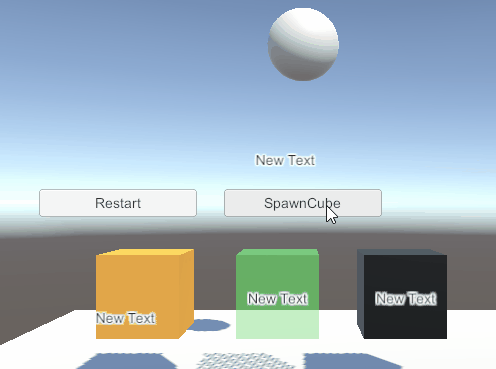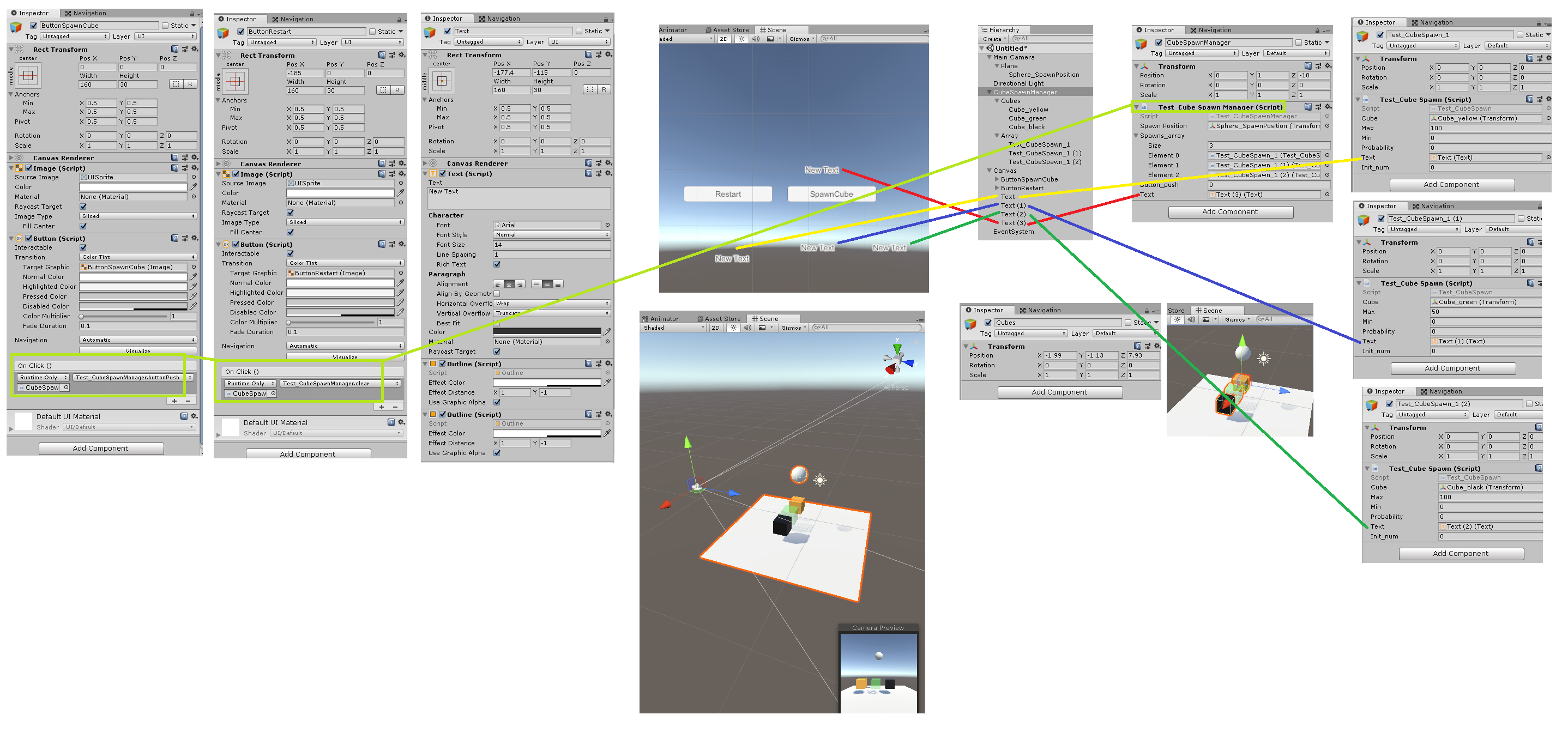# [Unity]随机装备属性随机数生成物品概率### 鉴定武器，新增武器属性的概率

int 武器稀有程度 = 100;//100为传说武器，90为绿色武器，80为蓝色武器，70为橘色武器，10为白色武器。

### 随机数占比数组 来生成 某些物品

...
int Probability_Max = 100;//最大数为100
int Probability_Monest_Lv1 = 60;//普通怪物
int Probability_Monest_Lv2 = 30;//精英怪物
int Probability_Monest_Lv3 = 10;//BOSS级别怪物
...


...
int Probability_Max = 100;//最大数为100
int Probability_Monest_Lv1 = 60;//普通怪物
int Probability_Monest_Lv2 = 30;//精英怪物
int Probability_Monest_Lv3 = 10;//BOSS级别怪物
int Probability_Monest_Lv4 = 10;//BOSS级别怪物
int Probability_Monest_Lv5 = 60;//普通怪物
int Probability_Monest_Lv6 = 30;//精英怪物
int Probability_Monest_Lv7 = 10;//BOSS级别怪物
int Probability_Monest_Lv8 = 10;//BOSS级别怪物
...


### 类似 WOW 里面，对 高级装备 提出需求的 摇骰子 的 随机数计算。100次，黄44，绿9，黑47

 黄 100 44 10 32 5 25 20 33 20 33 15 25 15 31 15 36 绿 50 9 10 33 5 29 20 31 20 32 15 40 15 33 15 22 黑 100 47 10 35 5 46 20 36 20 36 15 35 15 36 15 42

 黄 10 32 5 25 20 33 20 33 15 25 15 31 15 36 绿 10 33 5 29 20 31 20 32 15 40 15 33 15 22 黑 10 35 5 46 20 36 20 36 15 35 15 36 15 42

 黄 100 44 2 24 10 39 10 43 10 46 10 38 绿 50 9 1 0 5 9 5 7 5 10 5 8 黑 100 47 2 76 10 52 10 50 10 44 10 54

 黄 10 19 10 23 10 11 2 13 2 6 4 21 4 14 绿 15 57 15 58 15 59 3 51 3 53 6 47 6 57 黑 10 24 10 19 10 30 2 36 2 41 4 32 4 29

20:60:20/10:50:40/Test_CubeSpawnManager.cs

using UnityEngine;
using UnityEngine.UI;//Text

public class Test_CubeSpawnManager : MonoBehaviour {
/// <summary>
/// 生成 物品 的位置
/// </summary>
public Transform spawnPosition;

/// <summary>
/// 随机数生成 的方块
/// </summary>
public Test_CubeSpawn[] spawns_array;

/// <summary>
/// 当前 要生成的  方块 的概率
/// </summary>
private int currentProbability = 0;
/// <summary>
/// 当前的要生成的方块
/// </summary>
private Test_CubeSpawn currentCubeSpawn = null;//当前只 生成1个 方块。
/// <summary>
/// 生成的方块
/// </summary>
Transform go;

/// <summary>
/// 按钮按下的次数
/// </summary>
public int button_push;
/// <summary>
/// 显示的 文本 的按钮按下的次数
/// </summary>
public Text text;

/// <summary>
/// 当按钮SpawnCube 按下 执行的 函数
/// </summary>
public void buttonPush()
{
if (spawns_array.Length >0)
{
clear();//重置 所有的条件
button_push++;//记录 按下按钮的次数
int _int;
for (int i=0; i < spawns_array.Length; i++)
{ _int = spawns_array[i].spawnCube();
currentProbability = (currentProbability > _int) ? currentProbability : _int;
//当 currentProbability 大于 _int 的时候，则 保持 当前概率。否则 更新 当前的 概率
currentCubeSpawn = (currentProbability > _int) ? currentCubeSpawn : spawns_array[i];
}
if (currentCubeSpawn != null
&& spawnPosition != null)
{
currentCubeSpawn.init_num += 1;
go = Transform.Instantiate(currentCubeSpawn.cube) as Transform;//生成方块
go.position = spawnPosition.position;//设置 生成方块 的位置
if (currentCubeSpawn.text != null)
{
currentCubeSpawn.text.text = currentCubeSpawn.init_num.ToString();//使得对应方块的文本显示对应的次数
}
}
if (text != null)
{
text.text = button_push.ToString();//显示 按下按钮的次数
}

}
}

/// <summary>
/// 按下 restart按钮
/// </summary>
public void clear()
{
if ( go != null)
{
currentCubeSpawn = null;
currentProbability = 0;
Destroy(go.gameObject);
}
}
}


Test_CubeSpawn.cs

using UnityEngine;
using UnityEngine.UI;//Text

public class Test_CubeSpawn : MonoBehaviour {

/// <summary>
/// 要生成的 方块。
/// </summary>
public Transform cube;
/// <summary>
/// 最大概率
/// </summary>
public int max = 100;
/// <summary>
/// 最小概率
/// </summary>
public int min = 0;
/// <summary>
/// 当前概率
/// </summary>
public int probability = 0;
/// <summary>
/// 显示 对应方块的 文本 的生成 次数
/// </summary>
public Text text;
/// <summary>
/// 生成的次数
/// </summary>
public int init_num = 0;

/// <summary>
///  返回 一个概率值。
/// </summary>
/// <returns>概率</returns>
public int spawnCube()
{
probability = Random.Range(min,max);
return probability;
}
}


09-06860
02-26105
01-03750
12-021458
09-161010
09-013781
02-101839
12-021057
01-095215
07-08332
07-291257
02-222410
05-073868
04-2189
05-111938
04-181610
01-122807
09-26442
08-31345

### “相关推荐”对你有帮助么？

•非常没帮助
•没帮助
•一般
•有帮助
•非常有帮助被折叠的  条评论 为什么被折叠?到【灌水乐园】发言¥2 ¥4 ¥6 ¥10 ¥20余额支付 (余额：-- )扫码支付获取中扫码支付点击重新获取扫码支付1.余额是钱包充值的虚拟货币，按照1:1的比例进行支付金额的抵扣。
2.余额无法直接购买下载，可以购买VIP、C币套餐、付费专栏及课程。余额充值